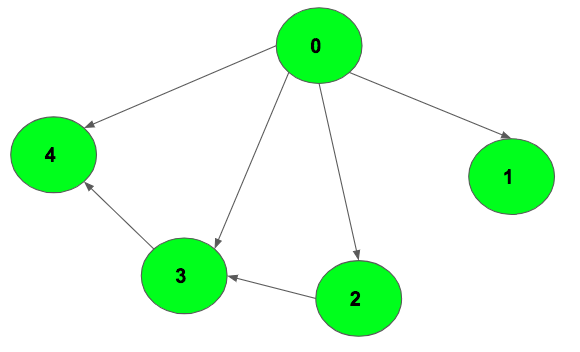# Number of paths from source to destination in a directed acyclic graph

Given a Directed Acyclic Graph with n vertices and m edges. The task is to find the number of different paths that exist from a source vertex to destination vertex.Examples:

Input: source = 0, destination = 4
Output: 3
Explanation:
0 -> 2 -> 3 -> 4
0 -> 3 -> 4
0 -> 4

Input: source = 0, destination = 1
Output: 1
Explanation: There exists only one path 0->1

## Recommended: Please try your approach on {IDE} first, before moving on to the solution.

Approach : Let f(u) be the number of ways one can travel from node u to destination vertex. Hence, f(source) is required answer. As f(destination) = 1 here so there is just one path from destination to itself. One can observe, f(u) depends on nothing other than the f values of all the nodes which are possible to travel from u. It makes sense because the number of different paths from u to the destination is the sum of all different paths from v1, v2, v3… v-n to destination vertex where v1 to v-n are all the vertices that have a direct path from vertex u. This approach, however, is too slow to be useful. Each function call branches out into further calls, and that branches into further calls, until each and every path is explored once.

The problem with this approach is the calculation of f(u) again and again each time the function is called with argument u. Since this problem exhibits both overlapping subproblems and optimal substructure, dynamic programming is applicable here. In order to evaluate f(u) for each u just once, evaluate f(v) for all v that can be visited from u before evaluating f(u). This condition is satisfied by reverse topological sorted order of the nodes of the graph.

Below is the implementation of the above approach:

 `// C++ program for Number of paths  ` `// from one vertex to another vertex ` `//  in a Directed Acyclic Graph ` `#include ` `using` `namespace` `std; ` `#define MAXN 1000005 ` ` `  `// to make graph ` `vector<``int``> v[MAXN]; ` ` `  `// function to add edge in graph ` `void` `add_edge(``int` `a, ``int` `b, ``int` `fre[]) ` `{ ` `    ``// there is path from a to b. ` `    ``v[a].push_back(b); ` `    ``fre[b]++; ` `} ` ` `  `// function to make topological sorting ` `vector<``int``> topological_sorting(``int` `fre[], ``int` `n) ` `{ ` `    ``queue<``int``> q; ` ` `  `    ``// insert all vertices which ` `    ``// don't have any parent. ` `    ``for` `(``int` `i = 0; i < n; i++) ` `        ``if` `(!fre[i]) ` `            ``q.push(i); ` ` `  `    ``vector<``int``> l; ` ` `  `    ``// using kahn's algorithm ` `    ``// for topological sorting ` `    ``while` `(!q.empty()) { ` `        ``int` `u = q.front(); ` `        ``q.pop(); ` ` `  `        ``// insert front element of queue to vector ` `        ``l.push_back(u); ` ` `  `        ``// go through all it's childs ` `        ``for` `(``int` `i = 0; i < v[u].size(); i++) { ` `            ``fre[v[u][i]]--; ` ` `  `            ``// whenever the freqency is zero then add ` `            ``// this vertex to queue. ` `            ``if` `(!fre[v[u][i]]) ` `                ``q.push(v[u][i]); ` `        ``} ` `    ``} ` `    ``return` `l; ` `} ` ` `  `// Function that returns the number of paths ` `int` `numberofPaths(``int` `source, ``int` `destination, ``int` `n, ``int` `fre[]) ` `{ ` ` `  `// make topological sorting ` `    ``vector<``int``> s = topological_sorting(fre, n); ` ` `  `    ``// to store required answer. ` `    ``int` `dp[n] = { 0 }; ` ` `  `    ``// answer from destination ` `    ``// to destination is 1. ` `    ``dp[destination] = 1; ` ` `  `    ``// traverse in reverse order ` `    ``for` `(``int` `i = s.size() - 1; i >= 0; i--) { ` `        ``for` `(``int` `j = 0; j < v[s[i]].size(); j++) { ` `            ``dp[s[i]] += dp[v[s[i]][j]]; ` `        ``} ` `    ``} ` ` `  `    ``return` `dp; ` `} ` ` `  `// Driver code ` `int` `main() ` `{ ` ` `  `    ``// here vertices are numbered from 0 to n-1. ` `    ``int` `n = 5; ` `    ``int` `source = 0, destination = 4; ` ` `  `    ``// to count number of vertex which don't ` `    ``// have any parents. ` `    ``int` `fre[n] = { 0 }; ` ` `  `    ``// to add all edges of graph ` `    ``add_edge(0, 1, fre); ` `    ``add_edge(0, 2, fre); ` `    ``add_edge(0, 3, fre); ` `    ``add_edge(0, 4, fre); ` `    ``add_edge(2, 3, fre); ` `    ``add_edge(3, 4, fre); ` ` `  `    ``// Function that returns the number of paths ` `    ``cout << numberofPaths(source, destination, n, fre); ` `} `

Output:

```3
```

My Personal Notes arrow_drop_uppawanasipugmailcom

If you like GeeksforGeeks and would like to contribute, you can also write an article using contribute.geeksforgeeks.org or mail your article to contribute@geeksforgeeks.org. See your article appearing on the GeeksforGeeks main page and help other Geeks.

Please Improve this article if you find anything incorrect by clicking on the "Improve Article" button below.